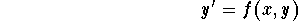#First Order Differential EquationsA first order differential equation is an equation involving the unknown function y, its derivative y' and the variable x. We will only talk about explicit differential equations[Geometry] [Algebra] [Trigonometry ]
[Calculus] [Complex Variables] [Matrix Algebra]S.O.S MATHematics home page

Do you need more help? Please post your question on our S.O.S. Mathematics CyberBoard.Author: Mohamed Amine Khamsi